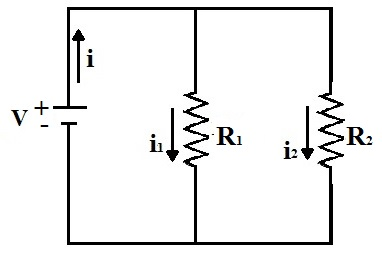Electricity & Megnetism

# Short circuit

A short circuit occurs when electric current flows through a conductor or device with negligible resistance, causing it to overheat.

Short circuits are so called because they represent the shortest path that electric current can take in a circuit. To better understand this phenomenon, we will make a detailed analysis of how a short circuit occurs.

The electrical circuit is the path that current travels between the two terminals of a voltage source. It is usually composed of an electricity generator and electrical resistances, so that when the circuit is closed, an electrical current is established between its terminals. Look at the following figure:An electrical circuit consists of a voltage source and electrical resistances in which an electrical current is established.

In the figure, the two resistors are in parallel. Therefore, the ddp across each resistor is equal to the source voltage (V). The electric current is proportional to the resistance in each resistor. If the two resistors are equal, then the electric current will be equal in the two resistors. But if we decrease the value of the resistance in R 1 , there will be an increase in the electric current passing through it and also in the electric current supplied by the source.

Considering now an extreme case, in which the value of R 1 is very close to the resistance of the circuit conductor, the electric current that passes through the resistor is very high, as well as the current that enters the circuit. The current in the other resistor, R 2 , is practically zero. This type of situation is what characterizes a short circuit.

“ A short circuit occurs when the electrical resistance in a circuit is very small and the electrical current flowing through it reaches a very high intensity.”

This increase in electrical current causes a large release of energy and, consequently, an overheating of the conductors. This heat release can be obtained mathematically.

First we use Ohm’s Law to relate the current (i) to the voltage (V) and resistance (R) of a circuit:

i = V
R

Then, we calculate the power dissipated in the resistor, which represents the amount of energy that is transformed into heat by the Joule effect, with the expression:

P = I

Substituting i, we have:

P = 2
R

From the equation obtained, we can conclude that the dissipated power is inversely proportional to the resistance value. Thus, the lower the resistance, the greater the dissipation of electrical energy in the conductor. Note that, in the equation above, if R tends to zero (R→0), P tends to infinity (P→∞).

The instantaneous dissipation of energy that occurs in a short circuit can generate sparks and explosions, causing several damages to electrical circuits, in addition to causing devastating fires in homes and industries. To avoid this type of accident, fuses and circuit breakers are used, which are devices that detect changes in the electrical current and automatically interrupt its passage.